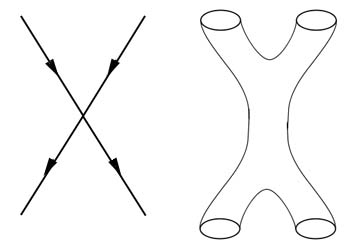## Saturday, July 28, 2012

### The Problems with Infinities

John D. Barrow in +plus magazine:
So infinities in modern physics have become separate from the study of infinities in mathematics. One area in physics where infinities are sometimes predicted to arise is aerodynamics or fluid mechanics. For example, you might have a wave becoming very, very steep and non-linear and then forming a shock. In the equations that describe the shock wave formation some quantities may become infinite. But when this happens you usually assume that it's just a failure of your model. You might have neglected to take account of friction or viscosity and once you include that into your equations the velocity gradient becomes finite — it might still be very steep, but the viscosity smoothes over the infinity in reality. In most areas of science, if you see an infinity, you assume that it's down to an inaccuracy or incompleteness of your model.Two particles meeting form a sharp corner (left) but two loops coming together are like two pairs of trousers sown together. (The trouser diagram has time going downwards and space horizontal.)
In particle physics there has been a much longer-standing and more subtle problem. Quantum electrodynamics is the best theory in the whole of science, its predictions are more accurate than anything else that we know about the Universe. Yet extracting those predictions presented an awkward problem: when you did a calculation to see what you should observe in an experiment you always seemed to get an infinite answer with an extra finite bit added on. If you then subtracted off the infinity, the finite part that you were left with was the prediction you expected to see in the lab. And this always matched experiment fantastically accurately. This process of removing the infinities was called renormalisation. Many famous physicists found it deeply unsatisfactory. They thought it might just be a symptom of a theory that could be improved.
This is why string theory created great excitement in the 1980s and why it suddenly became investigated by a huge number of physicists. It was the first time that particle physicists found a finite theory, a theory which didn't have these infinities popping up. The way it did it was to replace the traditional notion that the most basic entities in the theory (for example photons or electrons) should be point-like objects that move through space and time and so trace out lines in spacetime. Instead, string theory considers the most basic entities to be lines, or little loops, which trace out tubes as they move. When you have two point-like particles moving through space and interacting, it's like two lines hitting one another and forming a sharp corner at the place where they meet. It's that sharp corner in the picture that's the source of the infinities in the description. But if you have two loops coming together, it's rather like two legs of a pair of trousers. Then two more loops move out from the interaction — that's like sewing another pair of trousers onto the first pair. What you get is a smooth transition. This was the reason why string theory was so appealing, it was the first finite theory of particle physics. (h/t 3qd)